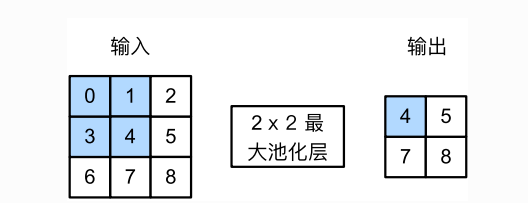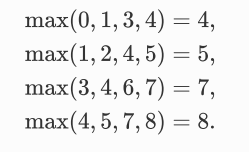# 深度学习|卷积神经网络之池化层

### 池化层

#### 二维最大池化层和平均池化层def pool2d(X, pool_size, mode='max'): # 默认模式参数为最大池化 'max'
X = X.float()
p_h, p_w = pool_size
Y = torch.zeros(X.shape - p_h + 1, X.shape - p_w + 1)
for i in range(Y.shape):
for j in range(Y.shape):
if mode == 'max':
Y[i, j] = X[i: i + p_h, j: j + p_w].max()
elif mode == 'avg':
Y[i, j] = X[i: i + p_h, j: j + p_w].mean()
return Y


X = torch.tensor([[0, 1, 2], [3, 4, 5], [6, 7, 8]])
pool2d(X, (2, 2)) # 默认‘max’模式 最大池化


tensor([[4., 5.],
[7., 8.]])


pool2d(X, (2, 2), 'avg')


tensor([[2., 3.],
[5., 6.]])

08-043万+07-2979
12-22690
03-255988
12-291105
02-183万+
11-075984
03-151530